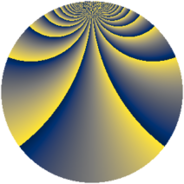# Properties

 Label 1104.2.bvLevel $1104$ Weight $2$ Character orbit 1104.bv Rep. character $\chi_{1104}(19,\cdot)$ Character field $\Q(\zeta_{44})$ Dimension $1920$ Sturm bound $384$

# Related objects

## Defining parameters

 Level: $$N$$ $$=$$ $$1104 = 2^{4} \cdot 3 \cdot 23$$ Weight: $$k$$ $$=$$ $$2$$ Character orbit: $$[\chi]$$ $$=$$ 1104.bv (of order $$44$$ and degree $$20$$) Character conductor: $$\operatorname{cond}(\chi)$$ $$=$$ $$368$$ Character field: $$\Q(\zeta_{44})$$ Sturm bound: $$384$$

## Dimensions

The following table gives the dimensions of various subspaces of $$M_{2}(1104, [\chi])$$.

Total New Old
Modular forms 3920 1920 2000
Cusp forms 3760 1920 1840
Eisenstein series 160 0 160

## Trace form

 $$1920q + 8q^{4} + O(q^{10})$$ $$1920q + 8q^{4} + 8q^{18} + 16q^{23} + 8q^{24} - 40q^{26} - 32q^{29} - 40q^{32} + 44q^{34} + 8q^{36} - 220q^{38} + 220q^{42} - 184q^{46} - 32q^{48} + 192q^{49} + 212q^{50} - 104q^{52} - 36q^{54} + 4q^{58} - 24q^{62} + 56q^{64} - 16q^{69} - 72q^{70} - 8q^{72} - 144q^{77} + 192q^{81} + 120q^{82} - 32q^{85} - 48q^{92} - 32q^{94} - 40q^{96} - 72q^{98} + O(q^{100})$$

## Decomposition of $$S_{2}^{\mathrm{new}}(1104, [\chi])$$ into newform subspaces

The newforms in this space have not yet been added to the LMFDB.

## Decomposition of $$S_{2}^{\mathrm{old}}(1104, [\chi])$$ into lower level spaces

$$S_{2}^{\mathrm{old}}(1104, [\chi]) \cong$$ $$S_{2}^{\mathrm{new}}(368, [\chi])$$$$^{\oplus 2}$$